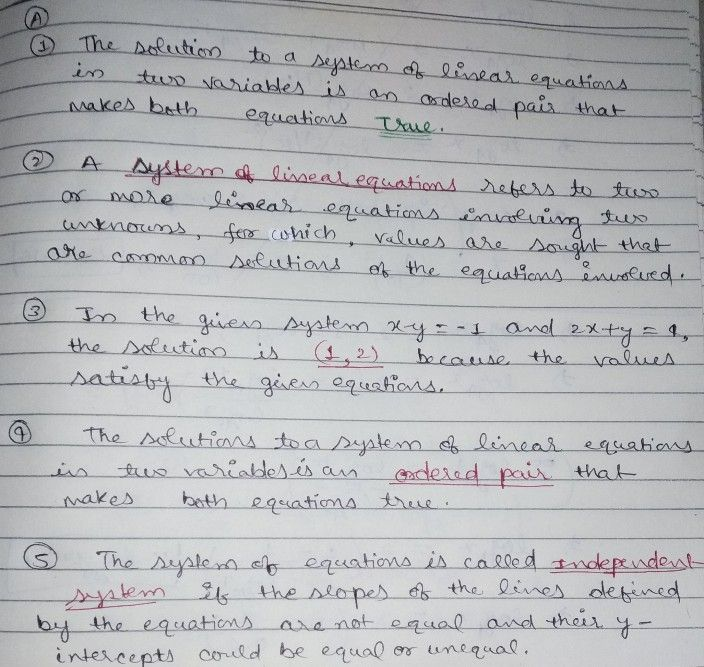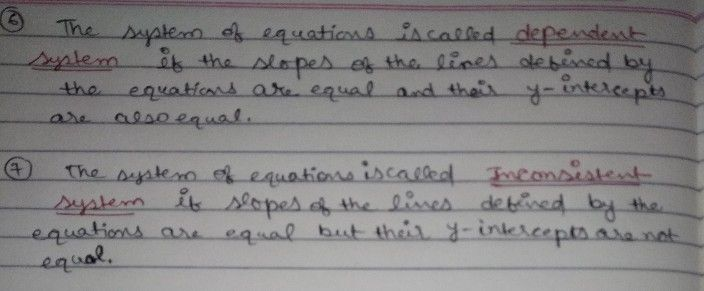Symbol
ProblemAdditional $Actis3t1c$ Complete Me! A Supply the correct term to $n11$ the $8a1cm<n1$ Write your answer on the blank. sorution to a $tcn$ of linear equations in two variables is an ordered pair that makes both equations 2. A $ctcrstotos0$ or more $l1n23\right)$ equations involving two $22nkna4n<$ for which, values are sought that are common $sa\left(o41c9s0rt\right)5$ equations involved. because the 3. In the given system $nx-y=-1$ $and2x+y=4$ the solution is values satisfy the given equations. 4. The solution to a system of linear equations in two variables is an that makes both equations true 5. The system of equations is $a1lc1$ if the $slc75$ of the lines defined by the equations are not equal and their $y=1n\left(ctcsP$ $c0111$ be equal or unequal. 6. The system of equations is $ca11c1$ if the slopes of the lines defined by the equations are equal and their $-1n1c\pi ccF$ are also equal. 7. The system of equations is called $fsiapc$ of the lines defined by the equations are equal but their $=1n1c$ $ccpt$ are not equal.
7th-9th grade
Calculus
Search count: 117
SolutionQanda teacher - Anjulihope it will help you.